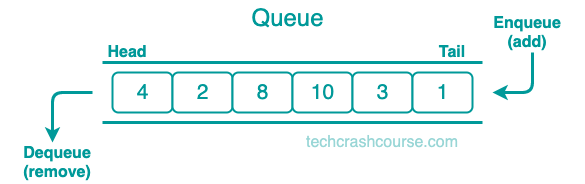# Queue Data Structure# Queue Data Structure

A Queue is a linear data structure that follows First-In-First-Out (FIFO) principle. This means that the first element added to the queue is the first element to be removed from the queue. Think of it like a line at a supermarket where the first person in line will be served first and so on.

The Queue data structure has two main operations - enqueue (add an element to the end of the queue) and dequeue (remove an element from the front of the queue). Additionally, there are two other important operations - peek (retrieve the element at the front of the queue without removing it) and isEmpty (check if the queue is empty)## Representation of a Queue

A Queue can be implemented using arrays and linked lists. In this tutorial, we will use arrays to implement the Queue. We will define a structure for the Queue that will have two variables: front and rear. The front variable will hold the index of the first element in the Queue, and the rear variable will hold the index of the last element in the Queue.

```struct Queue {
int front, rear;
int arr[MAXSIZE];
};
```
MAXSIZE is the maximum size of the Queue. It is the maximum number of elements a queue can store.

## Here's the Step-by-Step Implementation of the Common Queue Operations

### Initialize : To Initialize a Queue

Before using the Queue, we need to initialize it. We will set the front and rear variables to -1, which will indicate that the Queue is empty.

```void initializeQueue(struct Queue* q) {
q->front = -1;
q->rear = -1;
}
```

### isEmpty : Check if the Queue is Empty or Not

To check if the Queue is empty, we will check if the front variable is equal to -1. If it is, then the Queue is empty.

```int isEmpty(struct Queue* q) {
return q->front == -1;
}
```

### isFull : Check if the Queue is Full

To check if the Queue is full, we will check if the rear variable is equal to MAXSIZE-1. If it is, then the Queue is full.

```int isFull(struct Queue* q) {
return q->rear == MAXSIZE-1;
}
```

### enqueue : Add an Element to the Queue

To add an element to the Queue, we will first check if the Queue is full. If it is, we cannot add any more elements. If the Queue is not full, we will increment the rear variable and add the new element to the Queue.

```void enqueue(struct Queue* q, int x) {
if (isFull(q)) {
printf("Queue is full.\n");
return;
}
q->rear++;
q->arr[q->rear] = x;
if (q->front == -1) {
q->front = 0;
}
}
```

### dequeue : Remove an Element from the Queue

To remove an element from the Queue, we will first check if the Queue is empty. If it is, we cannot remove any element. If the Queue is not empty, we will remove the element at the front of the Queue and increment the front variable.

```int dequeue(struct Queue* q) {
if (isEmpty(q)) {
printf("Queue is empty.\n");
return -1;
}
int x = q->arr[q->front];
q->front++;
if (q->front > q->rear) {
q->front = q->rear = -1;
}
return x;
}
```

### printQueue : Display the Elements of the Queue

To display the elements of the Queue, we will loop through the Queue and print the elements.

```void display(struct Queue * q) {
if (isEmpty(q)) {
printf("Queue is empty.\n");
} else {
printf("Elements of Queue are: ");
for (int i = q - > front; i <= q - > rear; i++) {
printf("%d ", q - > arr[i]);
}
printf("\n");
}
}
```

## Array Implementation of Queue Data Structure

```#include <stdio.h>
#include <stdlib.h>

#define MAXSIZE 5

struct Queue {
int front, rear;
int arr[MAXSIZE];
};

void initializeQueue(struct Queue * q) {
q->front = -1;
q->rear = -1;
}

int isEmpty(struct Queue * q) {
return q->front == -1;
}

int isFull(struct Queue * q) {
return q->rear == MAXSIZE - 1;
}

void enqueue(struct Queue * q, int x) {
if (isFull(q)) {
printf("Queue is full.\n");
return;
}
q->rear++;
q->arr[q->rear] = x;
if (q->front == -1) {
q->front = 0;
}
}

int dequeue(struct Queue * q) {
if (isEmpty(q)) {
printf("Queue is empty.\n");
return -1;
}
int x = q->arr[q->front];
q->front++;
if (q->front > q->rear) {
q->front = q->rear = -1;
}
return x;
}

void display(struct Queue * q) {
if (isEmpty(q)) {
printf("Queue is empty.\n");
} else {
printf("Elements of Queue are: ");
for (int i = q->front; i <= q->rear; i++) {
printf("%d ", q->arr[i]);
}
printf("\n");
}
}

int main() {
struct Queue q;

initializeQueue(&q);

enqueue(&q, 10);
enqueue(&q, 20);
enqueue(&q, 30);
enqueue(&q, 40);
enqueue(&q, 50);
enqueue(&q, 60); // Queue is full.

// Elements of Queue are: 10 20 30 40 50
display(&q);

// Dequeued element: 10
printf("Dequeued element: %d\n", dequeue(&q));
// Dequeued element: 20
printf("Dequeued element: %d\n", dequeue(&q));

display(&q); // Elements of Queue are: 30 40 50

return 0;
}
```
Output
```Queue is full.
Elements of Queue are: 10 20 30 40 50
Dequeued element: 10
Dequeued element: 20
Elements of Queue are: 30 40 50
```

In the above program, we have defined a Queue with a maximum size of 5 elements. We have added 6 elements to the Queue, and as the Queue is full, we cannot add any more elements. We have then displayed the elements of the Queue, and we have dequeued the first two elements. We have again displayed the elements of the Queue.